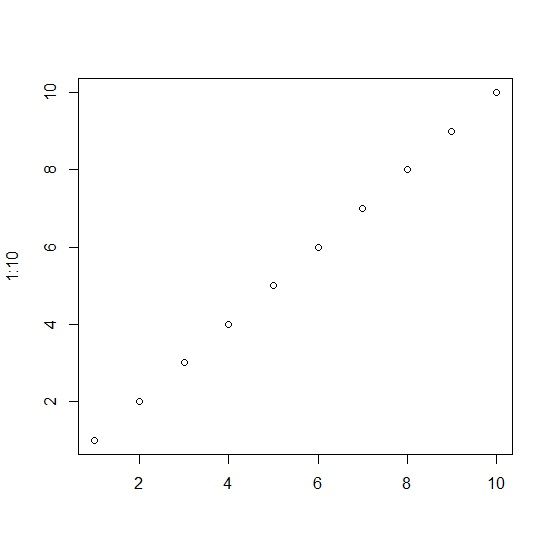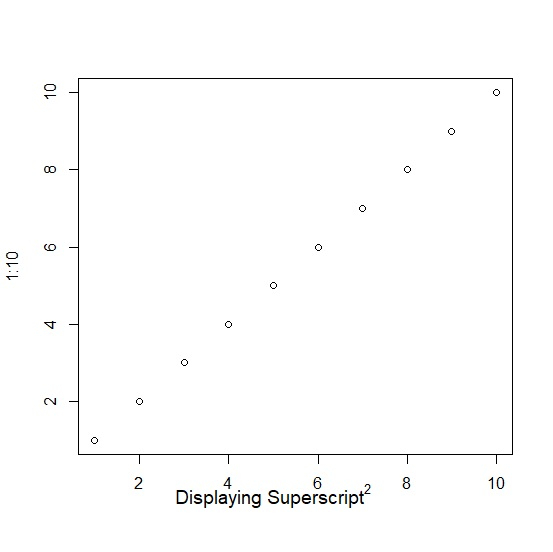# How to display superscript for X-axis title in base R plot?

To display superscript for X-axis title in base R plot, we can use ^ sign inside mtext function before defining the plain text.

For example, if we want to display X2 at position 5 on X-axis then it can be done by using the below command −

mtext(expression(paste(plain("X")^plain("2"))),side=1,line=2,at=5,cex=1.2)

## Example

Consider the following snippet −

plot(1:10)


## Output

If you execute the above given snippet, it generates the following Output −To display superscript for X-axis title in base R plot, add the following code to the above snippet −

## Example

plot(1:10,xlab="")

## Output

If you execute all the above given snippets as a single program, it generates the following Output −To display superscript for X-axis title in base R plot, add the following code to the above snippet −

## Example

mtext(expression(paste(plain("Displaying Superscript")^plain("2"))),side=1,line=2,at=5,cex=1.2)


## Output

If you execute all the above given snippets as a single program, it generates the following Output −